Imaginary numbers

Find two imaginary numbers whose sum is a real number. How are the two imaginary numbers related?
What is its sum?

Result

Sum =  0

Solution:

Answer is a conjugate pair of imaginary numbers (its real parts is zero).
The two imaginary numbers that add up to a real number would be ni and -ni, because ni + (-ni) = ni - ni = 0; where 'n' is any real number, no zero. Thus, the sum of this two imaginary numbers become a real number 0.Leave us a comment of example and its solution (i.e. if it is still somewhat unclear...):Be the first to comment!To solve this example are needed these knowledge from mathematics:

Try our complex numbers calculator.

Next similar examples:

1. Linear imaginary equationGiven that ? "this is z star" Find the value of the complex number z.
2. Is complexAre these numbers 2i, 4i, 2i + 1, 8i, 2i + 3, 4 + 7i, 8i, 8i + 4, 5i, 6i, 3i complex?
3. Complex number coordinatesWhich coordinates show the location of -2+3i
4. Im>0?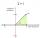Is -10i a positive number?
5. Median and modusRadka made 50 throws with a dice. The table saw fit individual dice's wall frequency: Wall Number: 1 2 3 4 5 6 frequency: 8 7 5 11 6 13 Calculate the modus and median of the wall numbers that Radka fell.
6. De Moivre's formula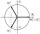There are two distinct complex numbers z such that z3 is equal to 1 and z is not equal 1. Calculate the sum of these two numbers.
7. ReciprocalCalculate reciprocal of z=0.8-1.8i:
8. SequenceBetween numbers 1 and 53 insert n members of the arithmetic sequence that its sum is 702.
9. Theorem proveWe want to prove the sentence: If the natural number n is divisible by six, then n is divisible by three. From what assumption we started?
10. Calculation of CNCalculate: ?
11. Sum of membersWhat is the sum of the first two members of the aritmetic progression if d = -4.3 and a3 = 7.5?
12. CalculationHow much is sum of square root of six and the square root of 225?
13. Linsys2Solve two equations with two unknowns: 400x+120y=147.2 350x+200y=144Combine like terms 4c+c-7c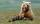Winnie Hugo had the right paw 10 punches and on 15 left more. How many punches has on left paw?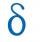Calculate the difference of arithmetic progression if the sum of its first 19 members Sn = 8075 and the first member is a1 = 20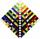Your task is express the sum of the following arithmetic series for n = 14: S(n) = 11 + 13 + 15 + 17 + ... + 2n+9 + 2n+11